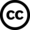## Faculty and Research Publications

Physics

Article

1-2018

7-3-2018

#### Abstract

We present the measurement of the absolute branching fractions of B+→Xc¯cK+ and B+→¯D(*)0π+decays, using a data sample of 772×106  B¯B pairs collected at the Υ(4S) resonance with the Belle detector at the KEKB asymmetric-energy e+e− collider. Here, Xc¯c denotes ηc, J/ψ, χc0, χc1, ηc(2S), ψ(2S), ψ(3770), X(3872), and X(3915). We do not observe significant signals for X(3872) or X(3915) and set the 90% confidence level upper limits at B(B+→X(3872)K+)<2.6×10−4 and B(B+→X(3915)K+)<2.8×10−4. These represent the most stringent upper limit for B(B+→X(3872)K+) to date and the first limit for B(B+→X(3915)K+). The measured branching fractions for ηc and ηc(2S) are the most precise to date, B(B+→ηcK+)=(12.0±0.8±0.7)×10−4and B(B+→ηc(2S)K+)=(4.8±1.1±0.3)×10−4, where the first and second uncertainties are statistical and systematic, respectively.

#### Journal Title

Physical Review D

2470-0010

97

1

#### Digital Object Identifier (DOI)

10.1103/PhysRevD.97.012005